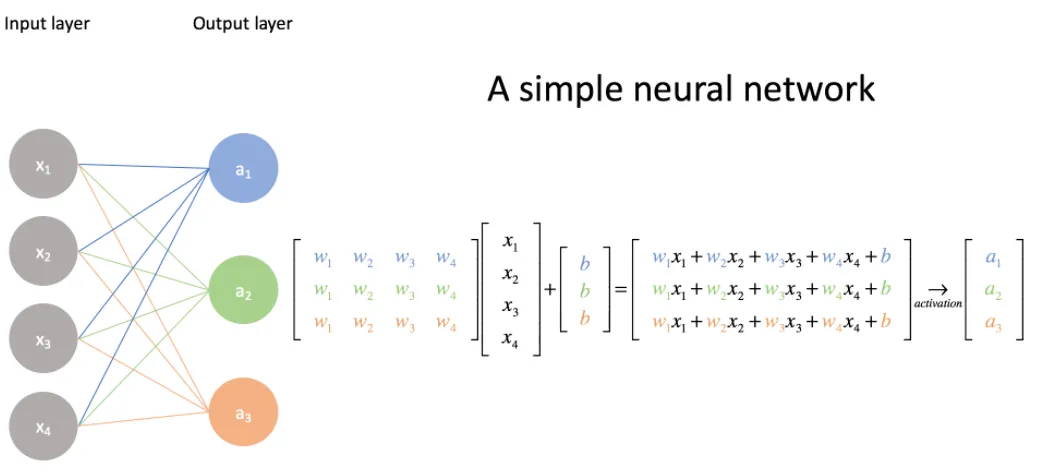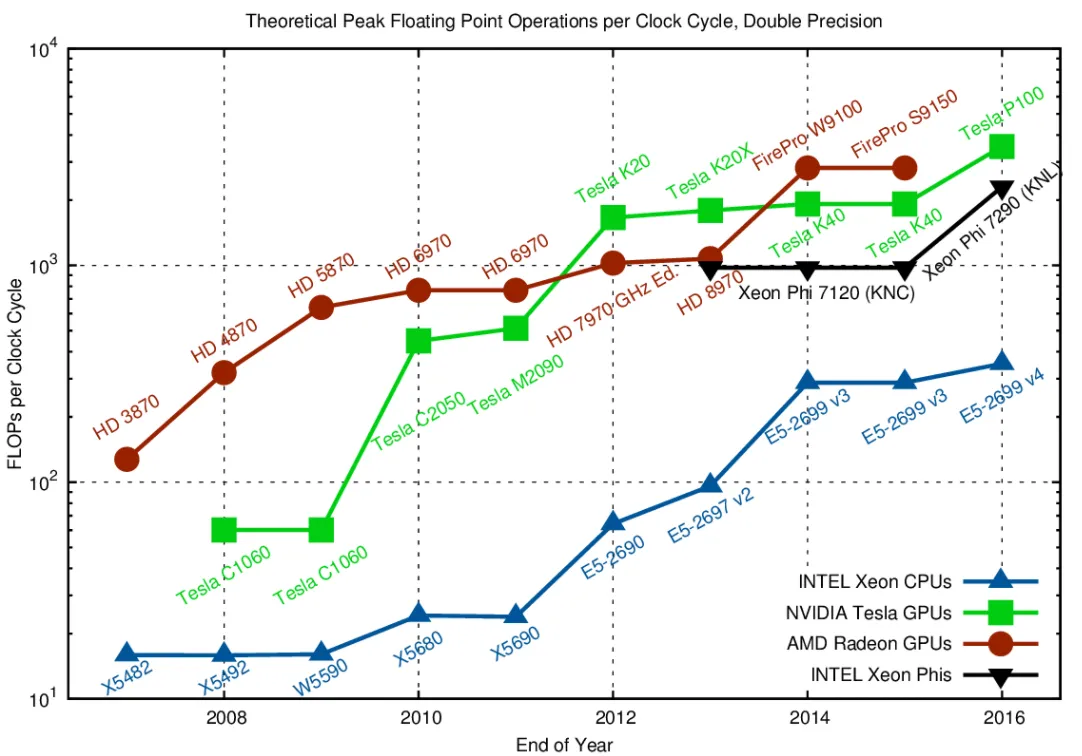# GPU

## What is a Graphics Processing Unit (GPU)?

A graphics processing unit (GPU) is a specialized circuit designed to rapidly manipulate and alter memory to accelerate computer graphics and image processing. Modern GPUs’ highly parallel structure makes them more efficient than central processing units (CPUs) for algorithms that process large blocks of data in parallel.

GPUs are considered essential to deep learning/neural networks. For any neural network, the training phase of the deep learning model is the most resource-intensive task. While training, a neural network takes in inputs, which are then processed in hidden layers using weights that are adjusted during training, and the model then outputs a prediction. Weights are adjusted to find patterns in order to make better predictions. These operations are essentially matrix multiplications. A simple matrix multiplication can be represented by the image below:Source: Jeremy Jordan

In a neural network, the first array is typically the input, while the second array represents weights. These calculations are relatively straightforward if your neural network has around 10, 100 or even 100,000 parameters. A computer would still be able to handle this in a matter of minutes, or even hours at the most.

But what if your neural network has more than 10 billion parameters? It would take years to train this kind of system with CPUs. Deep learning models can be trained much faster by running all operations at the same time instead of sequentially. GPUs are optimized for training deep learning models, as they can process multiple computations simultaneously.

While CPUs are mostly applicable for problems that require parsing through or interpreting complex logic in code, GPUs are designed to be the dedicated graphical rendering workhorses of computer games. GPUs have been enhanced to accelerate other geometric calculations involving matrix multiplication. They devote proportionally more transistors to arithmetic logic units and fewer to caches and flow control as compared to CPUs.

## Floating Point Operations Much Faster with GPUs vs CPUsSource: fast.ai